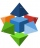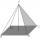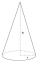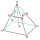# Pyramid + quadrilateral - math problems

#### Number of problems found: 42

• Special bodyAbove each wall of a cube with an edge a = 30 cm, a regular quadrilateral pyramid with a height of 15 cm is constructed. Find the volume of the resulting body.
• Metal pyramidFind the weight of a regular quadrilateral pyramid with a 5 cm length and 6,5 cm body height is made from material with density g/cm3.
• The castThe cast in the body of a regular quadrilateral pyramid with a base edge 60 cm long and 5 cm high is made of a material with a density of 7.8 g/cm cubic. Calculate its weight.In a regular quadrilateral pyramid, the height is 6.5 cm and the angle between the base and the side wall is 42°. Calculate the surface area and volume of the body. Round calculations to 1 decimal place.How many square meters are needed to cover the shape of a regular quadrangular pyramid base edge 10 meters if the deviation lateral edges from the base plane are 68°? Calculate waste 10%.A quadrilateral pyramid, which has a rectangular base with dimensions of 24 cm, 13 cm. The height of the pyramid is 18cm. Calculate 1/the area of the base 2/casing area 3/pyramid surface 4/volume of the pyramid
• Tetrahedral pyramidA regular tetrahedral pyramid is given. Base edge length a = 6.5 cm, side edge s = 7.5 cm. Calculate the volume and the area of its face (side area).
• From plasticineMichael modeled from plasticine a 15 cm high pyramid with a rectangular base with the sides of the base a = 12 cm and b = 8 cm. From this pyramid, Janka modeled a rotating cone with a base diameter d = 10 cm. How tall was Janka's cone?
• Digging a pitThe pit has the shape of a regular quadrilateral truncated pyramid. The edges of the bases are 14m and 10m long. The sidewalls form an angle of 135° with a smaller base. Determine how many m3 of soil were excavated when digging the pit?
• Tetrahedral pyramidCalculate the regular tetrahedral pyramid's volume and surface if the content area of the base is 20 cm2, and the deviation angle of the side edges from the plane of the base is 60 degrees.
• School modelThe beech school model of a regular quadrilateral pyramid has a base 20 cm long and 24 cm high. Calculate a) the surface of the pyramid in square decimeters, b) the mass of the pyramid in kilograms if the density of the beech is ρ = 0,8 g/cm ^ 3
• Secret treasureScouts have a tent in the shape of a regular quadrilateral pyramid with a side of the base 4 m and a height of 3 m. Find the container's radius r (and height h) so that they can hide the largest possible treasure.
• Truncated pyramidThe truncated regular quadrilateral pyramid has a volume of 74 cm3, a height v = 6 cm, and an area of the lower base 15 cm2 greater than the upper base's content. Calculate the area of the upper base.The regular quadrilateral pyramid has a base edge a = 1.56 dm and a height h = 2.05 dm. Calculate: a) the deviation angle of the sidewall plane from the base plane b) deviation angle of the side edge from the plane of the base
• Truncated pyramidThe concrete pedestal in the shape of a regular quadrilateral truncated pyramid has a height of 12 cm, the pedestal edges have lengths of 2.4 and 1.6 dm. Calculate the surface of the base.
• Truncated pyramidFind the volume and surface area of a regular quadrilateral truncated pyramid if base lengths a1 = 17 cm, a2 = 5 cm, height v = 8 cm.
• 4side pyramidCalculate the volume and surface of the regular four-sided pyramid whose base edge is 4 cm long. The angle from the plane of the sidewall and base plane is 60 degrees.We have a regular quadrilateral pyramid with a base edge a = 10 cm and a height v = 7 cm. Calculate 1/base content 2/casing content 3/pyramid surface 4/volume of the pyramidFind the surface area of a regular quadrilateral pyramid if for its volume V and body height v and the base edge a applies: V = 2.8 m ^ 3, v = 2.1 mThe quadrilateral pyramid has a rectangular base of 24 cm x 3.2dm and a body height of 0.4m. Calculate its volume and surface area.